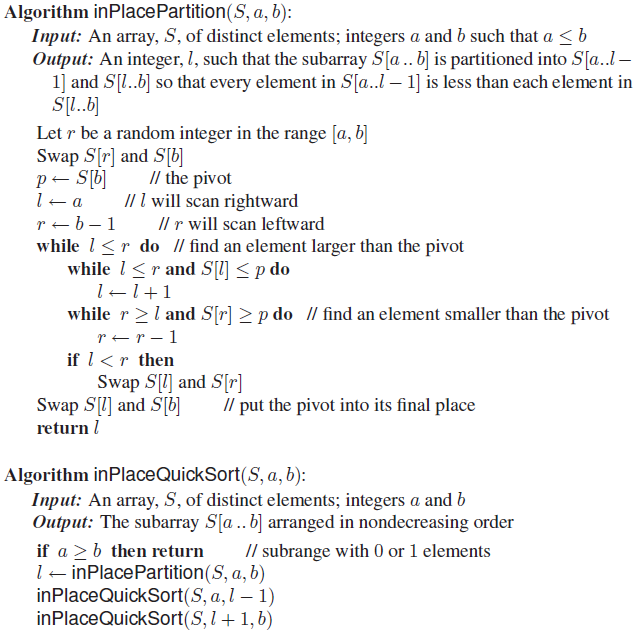# Answered Essay: Algorithm inPlacePartition(S, a, b): Input: An array, S, of distinct elements: integers a and b such that a lessthanorequ

Prove this algorithm for in-place quicksort is correct using induction. Also include the loop invariant proof:Algorithm inPlacePartition(S, a, b): Input: An array, S, of distinct elements: integers a and b such that a lessthanorequalto b Output: An integer, l, such that the subarray S[a b] is partitioned into S[a..l – 1] and S[l..b] so that every element in S[a..l – 1] is less than each element in S[l..b] Let r be a random integer in the range [a, b] Swap S[r] and S[b] p leftarrow S[b] // the pivot l leftarrow a // l will scan rightward r leftarrow b – 1 // r will scan leftward while l lessthanorequalto r do // find an element larger than the pivot while l lessthanorequalto r and S[l] lessthanorequalto p do l leftarrow l + 1 while r greaterthanorequalto l and S[r] greaterthanorequalto p do // find an element smaller than the pivot r leftarrow r – 1 if l

Base Condition:

If n = 1, Since only one element is there the algorithm is correct.

Induction Hypothesis:

It is correct on lists of size smaller than n. So algorithm is correct for list smaller than size n .

Inductive step:

After positioning, the pivot p at position i; i = 1, . . . , n − 1, splits a list of size n into the head sublist of size i and the tail sublist of size n − 1 − i. Elements of the head sublist are not greater than p. Elements of the tail sublist are not smaller than p. • By the induction hypothesis, both the head and tail sublists are sorted correctly. • Therefore, the whole list of size n is sorted correctly.

———————————————————————————————————————————————————

We will prove the correctness using loop invariant.

Let (S, a, b) be any input to inPlacePartition and let c be the output of Partition on this input. Suppose 1 ≤ a < b.

Let x = A[b].

at the beginning of the for-loop, for all k, a ≤ k ≤ b, the following properties hold:

1. If a ≤ k ≤ i, then A[k] ≤ x.

2. If i + 1 ≤ k ≤ j − 1, then A[k] > x.

3. If k = b, then A[k] = x

Initialization:

The initial value of i is a − 1 and the initial value of j is a. So, there is no k such a ≤ k ≤ i and there is no k such that

i + 1 ≤ k ≤ j − 1. Thus, the first conditions are met. The initial value of A[b] = x, is so the last one is met.

Maintenance:

Suppose that the three conditions are met at the beginning and that j ≤ b − 1.

Suppose that A[j] > x. The value of i will not be changed, so (1) holds. The value of j becomes j + 1. Since A[j] > x, (2) will for the new value of j. Also, A[b] is unchanged so (3) holds. Suppose that A[j] ≤ x. Then A[i + 1] and A[j] will be exchanged. By (2), A[i + 1] > x. So, after exchange A[i + 1] ≤ x and A[j] > x. Both i and j will be incremented by 1, so (1) and (2) will be preserved. Again (3) still holds.

Termination:

At the end, j = b. So, for all k, 1 ≤ k ≤ i, A[k] ≤ x and for all k, i + 1 ≤ k ≤ b − 1, A[k] > x.

So the Quick Sort algorithm is proved to be correct in loop entrance , while in the loop and after termination of the loop.Hence Proved.Calculate your paper price
Pages (550 words)
Approximate price: -

Help Me Write My Essay - Reasons:Best Online Essay Writing Service

We strive to give our customers the best online essay writing experience. We Make sure essays are submitted on time and all the instructions are followed.Our Writers are Experienced and Professional

Our essay writing service is founded on professional writers who are on stand by to help you any time.Free Revision Fo all Essays

Sometimes you may require our writers to add on a point to make your essay as customised as possible, we will give you unlimited times to do this. And we will do it for free.Timely Essay(s)

We understand the frustrations that comes with late essays and our writers are extra careful to not violate this term. Our support team is always engauging our writers to help you have your essay ahead of time.Customised Essays &100% Confidential

Our Online writing Service has zero torelance for plagiarised papers. We have plagiarism checking tool that generate plagiarism reports just to make sure you are satisfied.Our agents are ready to help you around the clock. Please feel free to reach out and enquire about anything.

Try it now!

## Calculate the price of your order

Total price:
\$0.00

How it works?

Follow these simple steps to get your paper doneFill in the order form and provide all details of your assignment.Proceed with the payment

Choose the payment system that suits you most.Receive the final file

Once your paper is ready, we will email it to you.

HOW OUR ONLINE ESSAY WRITING SERVICE WORKS

Let us write that nagging essay.## Submit Your Essay/Homework Instructions

By clicking on the "PLACE ORDER" button, tell us your requires. Be precise for an accurate customised essay. You may also upload any reading materials where applicable.Pick A & Writer

Our ordering form will provide you with a list of writers and their feedbacks. At step 2, its time select a writer. Our online agents are on stand by to help you just in case.Editing (OUR PART)

At this stage, our editor will go through your essay and make sure your writer did meet all the instructions.